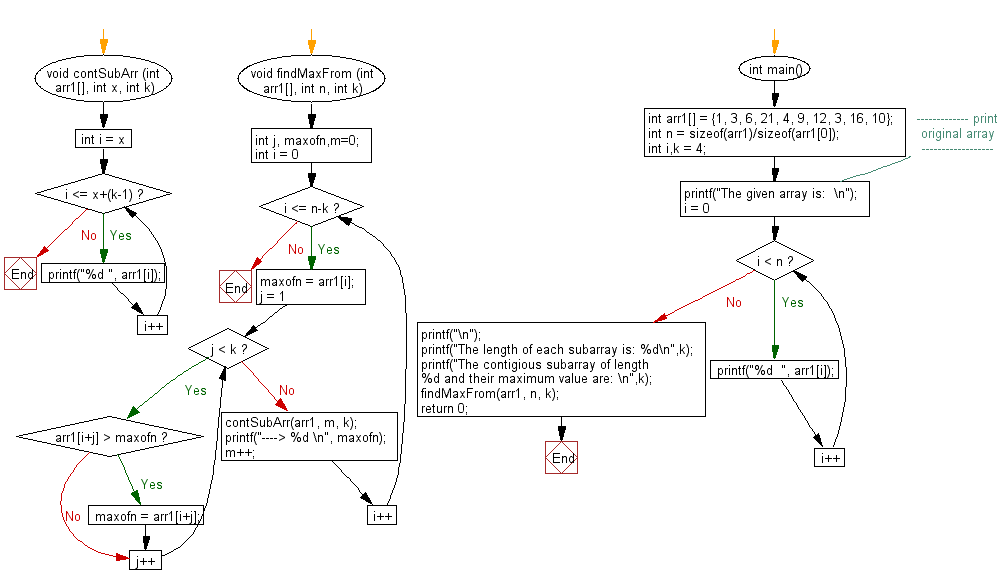﻿ C exercises: Find the maximum for each and every contigious subarray of size k from a given array - w3resource# C Exercises: Find the maximum for each and every contigious subarray of size k from a given array

## C Array: Exercise-94 with Solution

Write a program in C to find the maximum for each and every contigious subarray of size k from a given array.

Sample Solution:

C Code:

``````#include<stdio.h>

void contSubArr (int arr1[], int x, int k)
{
for (int i = x; i <= x+(k-1); i++)
{
printf("%d ", arr1[i]);
}
}

void findMaxFrom (int arr1[], int n, int k)
{
int j, maxofn,m=0;
for (int i = 0; i <= n-k; i++)
{
maxofn = arr1[i];

for (j = 1; j < k; j++)
{
if (arr1[i+j] > maxofn)
maxofn = arr1[i+j];
}
contSubArr(arr1, m, k);
printf("----> %d \n", maxofn);
m++;
}
}

int main()
{
int arr1[] = {1, 3, 6, 21, 4, 9, 12, 3, 16, 10};
int n = sizeof(arr1)/sizeof(arr1);
int i,k = 4;
//------------- print original array ------------------
printf("The given array is:  \n");
for(i = 0; i < n; i++)
{
printf("%d  ", arr1[i]);
}
printf("\n");
//-----------------------------------------------------------
printf("The length of each subarray is: %d\n",k);
printf("The contigious subarray of length %d and their maximum value are: \n",k);
findMaxFrom(arr1, n, k);
return 0;
}
```
```

Sample Output:

```The given array is:
1  3  6  21  4  9  12  3  16  10
The length of each subarray is: 4
The contigious subarray of length 4 and their maximum value are:
1 3 6 21 ----> 21
3 6 21 4 ----> 21
6 21 4 9 ----> 21
21 4 9 12 ----> 21
4 9 12 3 ----> 12
9 12 3 16 ----> 16
12 3 16 10 ----> 16
```

Pictorial Presentation:Flowchart:C Programming Code Editor:

Improve this sample solution and post your code through Disqus.

﻿